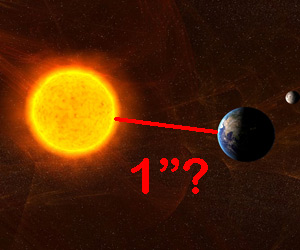# BAFact math: Give him an inch and he’ll take a light year[BAFacts are short, tweetable astronomy/space facts that I post every day. On some occasions, they wind up needing a bit of a mathematical explanation. The math is pretty easy, and it adds a lot of coolness, which I’m passing on to you! You’re welcome.]

Today’s BAFact: To scale, if the Earth/Sun distance were one inch, a light year would be exactly one mile.Humans have a miserable sense of scale. Space is huge - that’s why we call it “space” - but how huge? Here’s a fun trick my friend Dan Durda pointed out to me many years ago when we were in college together (forgive my not using metric units, but what the heck, this only works in imperial):

The average distance of the Earth to the Sun (what we call an astronomical unit) is about 92.8 million miles. If you made a scale model of the solar system where that distance were equal to one inch, then one mile in the model would be almost exactly a light year in the real world!

The math is easy. One light year is the distance light travels in a year. The speed of light is 186,282 miles/second, and distance equals speed multiplied by time. So:

186,282 mi/sec x 86,400 sec/day x 365.25 days/year = 5.88 trillion miles

[Note: I’m rounding the answer to two decimal places for ease of comparison.]

OK, now what about our scale? There are 12 inches to a foot, and 5280 feet to a mile. That means there are

12 in/ft x 5280 ft/mile = 63,360 inches/mile

If we let 1 inch = 92.8 million miles, then 63,360 inches = 5.88 trillion miles.

See? To two decimal places the scale is exact! In real life the Earth orbits the Sun in an ellipse, so there’s a roughly 3% change in distance over time. But if we just take the average distance, this works perfectly.

So the next time you’re out driving, keep that in mind… The nearest known star to the Sun is Proxima Centauri, roughly 4.2 light years away. That means it’s 4.2 miles away to scale, and at 60 mph would take over four minutes to reach. At that same speed, you’re crossing the entire Earth/Sun distance in less than one-thousandth of second!

If you really tried to drive from the Earth to the Sun (and there were a heavenly highway connecting them) at that speed it would take over 175 years.

From a scale model millisecond to more than a century. Did I mention space is big?

Original image credit: sebikus/shutterstock.com

Related Posts: